Courses

# Chapter Notes - Symmetry Class 6 Notes | EduRev

## Class 6 : Chapter Notes - Symmetry Class 6 Notes | EduRev

The document Chapter Notes - Symmetry Class 6 Notes | EduRev is a part of the Class 6 Course Mathematics (Maths) Class 6.
All you need of Class 6 at this link: Class 6

13. Symmetry

Line Symmetry: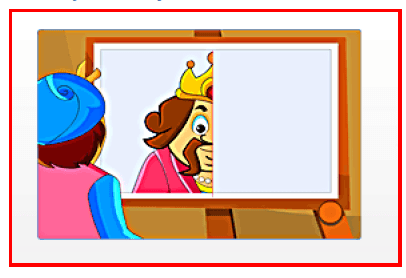A figure can have line symmetry if a line can be drawn dividing it into two equal halves. The line is called the line of symmetry.

A figure can have line symmetry if a line can be drawn dividing it into two equal halves. The line is called the line of symmetry. We can find examples of objects showing line symmetry in nature. For example, a butterfly, some leaves and flowers show line symmetry.

Examples of line symmetry can also be found in many of our ancient and modern buildings.

Objects that show line symmetry appear more balanced and beautiful.

A kite shape has only one line of symmetry.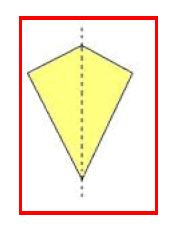A rectangle has two lines of symmetry.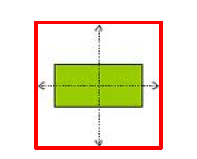An equilateral triangle has three lines of symmetry.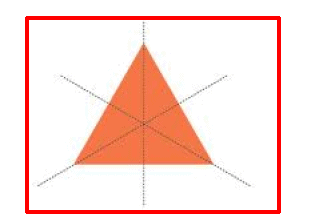Page 55

A circle has an infinite number of lines of symmetry.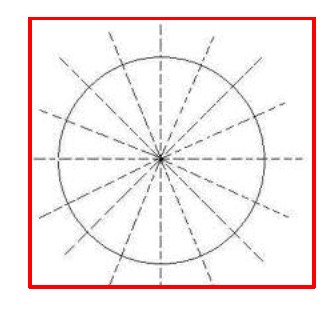A shape may have just one or more than one lines of symmetry. When completing a given figure against a given line of symmetry, make sure that:
Each part of the constructed figure is equal in measurement to its corresponding part in the given figure.
Each point on the given figure and its corresponding point on the constructed figure are at the same distance from the line of symmetry.

Mirror Symmetry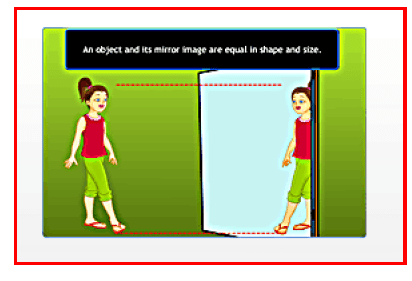The line of symmetry is related to mirror reflection.

Note Page 56

The line of symmetry is related to mirror reflection. An object and its mirror image are equal in shape and size. An object and its image are always at the same distance from the surface of a mirror, which is called the mirror line.

The left and the right sides of an object appear inverted in a mirror. An object and its image show mirror symmetry, with the mirror line being the line of symmetry.

Letters written from right to left, appear written from left to right in their mirror image. Letters like A, M and U appear the same in their mirror image. The letters A, H, I, M, O, T, U, V, W, X and Y appear the same in their mirror image.

All the other letters of the alphabet appear reversed in their mirror image. Symmetry has plenty of applications in real life, as in art, architecture, textiles designing, geometrical reasoning, Kolams, Rangoli, etc.

Offer running on EduRev: Apply code STAYHOME200 to get INR 200 off on our premium plan EduRev Infinity!

## Mathematics (Maths) Class 6

191 videos|220 docs|43 tests

,

,

,

,

,

,

,

,

,

,

,

,

,

,

,

,

,

,

,

,

,

;